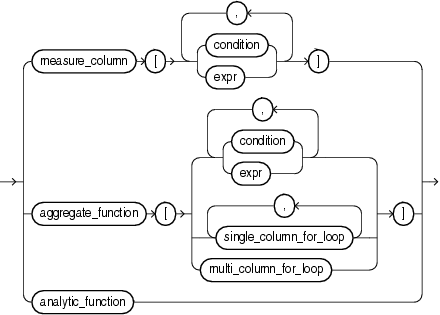# Model Expressions

A model expression is used only in the `model_clause` of a `SELECT` statement and then only on the right-hand side of a model rule. It yields a value for a cell in a measure column previously defined in the `model_clause`. For additional information, refer to model_clause .

model_expression::=Description of the illustration model_expression.gif

When you specify a measure column in a model expression, any conditions and expressions you specify must resolve to single values.

When you specify an aggregate function in a model expression, the argument to the function is a measure column that has been previously defined in the `model_clause`. An aggregate function can be used only on the right-hand side of a model rule.

Specifying an analytic function on the right-hand side of the model rule lets you express complex calculations directly in the `model_clause`. The following restrictions apply when using an analytic function in a model expression:

• Analytic functions can be used only in an `UPDATE` rule.

• You cannot specify an analytic function on the right-hand side of the model rule if the left-hand side of the rule contains a `FOR` loop or an `ORDER` `BY` clause.

• The arguments in the `OVER` clause of the analytic function cannot contain an aggregate.

• The arguments before the `OVER` clause of the analytic function cannot contain a cell reference.

"The MODEL clause: Examples" for an example of using an analytic function on the right-hand side of a model rule

When `expr` is itself a model expression, it is referred to as a nested cell reference. The following restrictions apply to nested cell references:

• Only one level of nesting is allowed.

• A nested cell reference must be a single-cell reference.

• When `AUTOMATIC` `ORDER` is specified in the `model_rules_clause`, a nested cell reference can be used on the left-hand side of a model rule only if the measure used in the nested cell reference is never updated for any cell in the spreadsheet clause.

The model expressions shown below are based on the `model_clause` of the following `SELECT` statement:

```SELECT country,prod,year,s
FROM sales_view_ref
MODEL
PARTITION BY (country)
DIMENSION BY (prod, year)
MEASURES (sale s)
IGNORE NAV
UNIQUE DIMENSION
RULES UPSERT SEQUENTIAL ORDER
(
s['Standard Mouse', 2001] = s['Standard Mouse', 2000]
)
ORDER BY country, prod, year;
```

The following model expression represents a single cell reference using symbolic notation. It represents the sales of the Mouse Pad for the year 2000.

```s[prod='Mouse Pad',year=2000]
```

The following model expression represents a multiple cell reference using positional notation, using the `CV` function. It represents the sales of the current value of the dimension column `prod` for the year 2001.

```s[CV(prod), 2001]
```

The following model expression represents an aggregate function. It represents the sum of sales of the Mouse Pad for the years between the current value of the dimension column `year` less two and the current value of the dimension column `year` less one.

```SUM(s)['Mouse Pad',year BETWEEN CV()-2 AND CV()-1]
```